# Python短代码练习与技巧_2

2021-10-18 334点热度 0人点赞 0条评论

# 你必须掌握的20个Python代码，短小精悍，用处无穷

from https://zhuanlan.zhihu.com/p/370071156 by 囍歡

## 1.字符串的翻转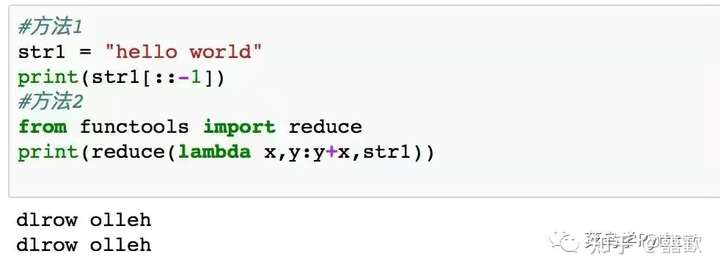## 2.判断字符串是否是回文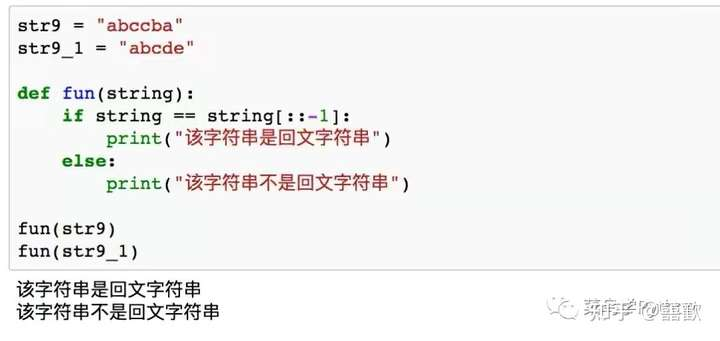## 3.单词大小写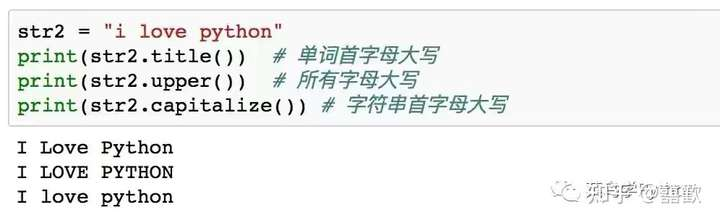## 4.字符串的拆分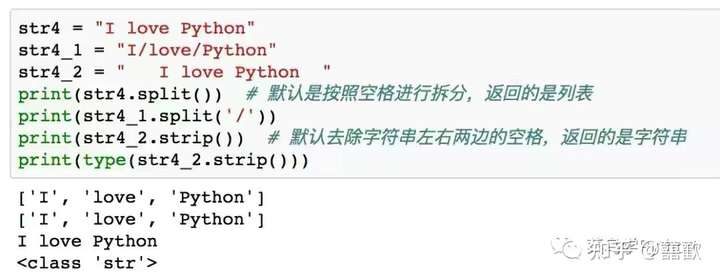## 5.将列表中的字符串合并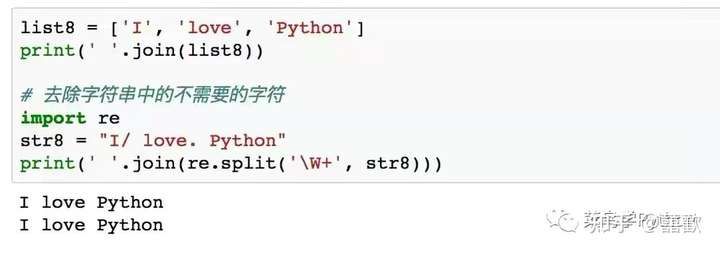## 6.寻找字符串中唯一的元素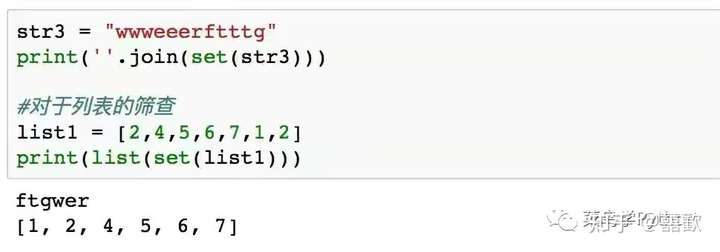## 7.将元素进行重复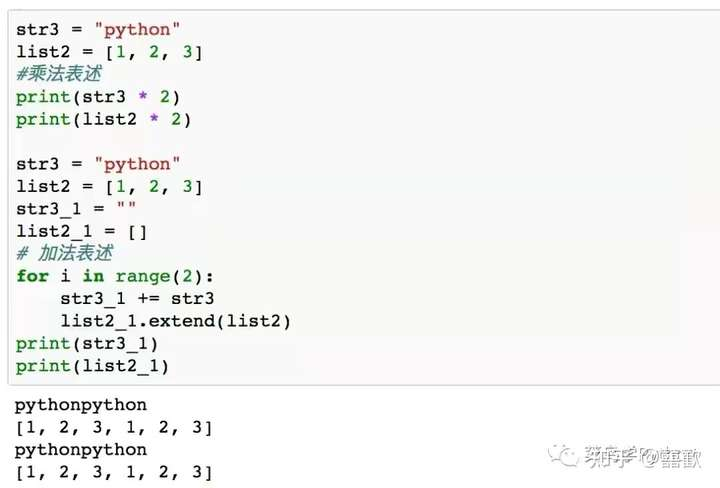## 8.基于列表的扩展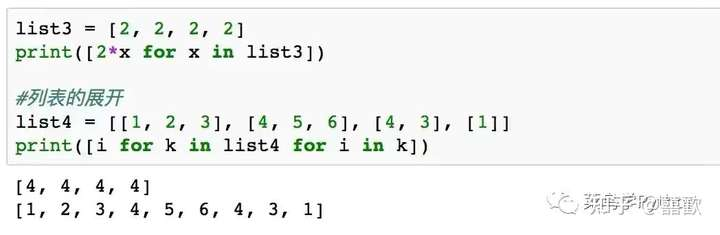## 9. 将列表展开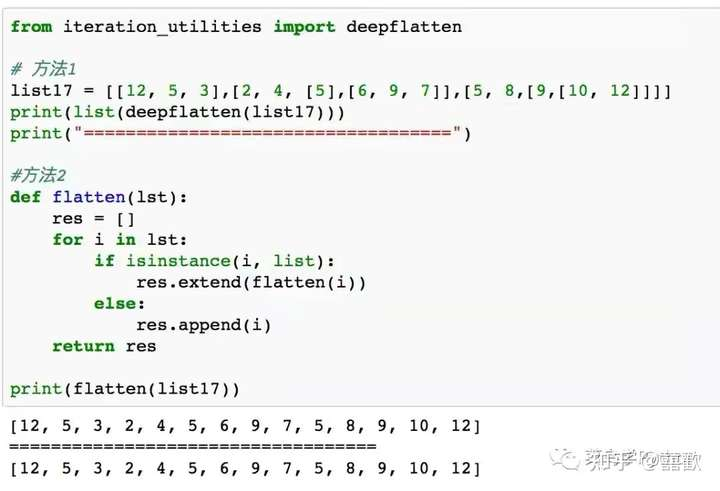## 10.二值交换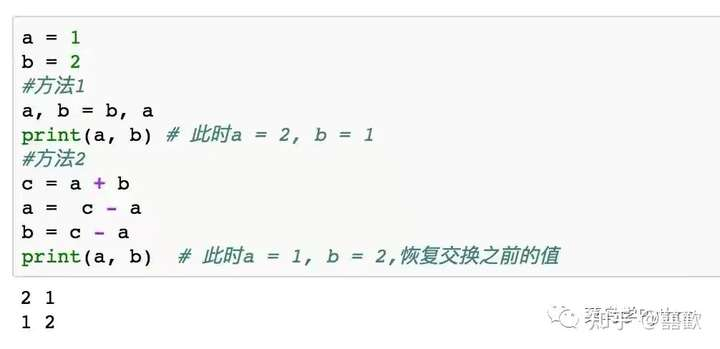Python中的二值交换，可以直接采用交换的方式，如上图的方法1，而方法2所示的方法，借助第三个变量，来实现了两个数值的交换。

## 11.统计列表中元素的频率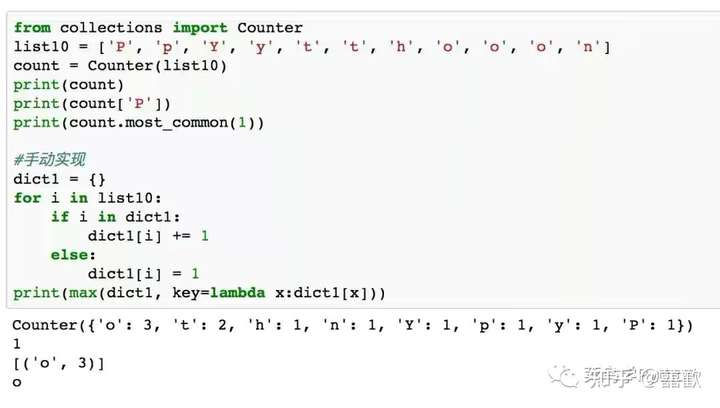## 12.判断字符串所含元素是否相同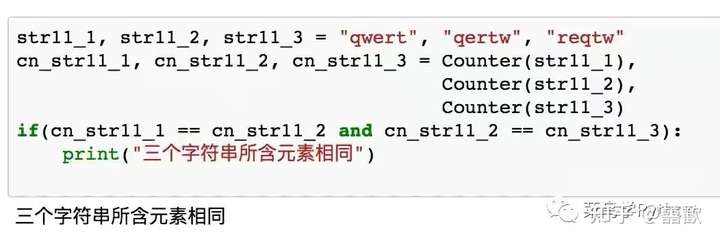Counter函数还可以用来判断字符串中包含的元素是否相同，无论字符串中元素顺序如何，只要包含相同的元素和数量，就认为其是相同的。

## 13.将数字字符串转化为数字列表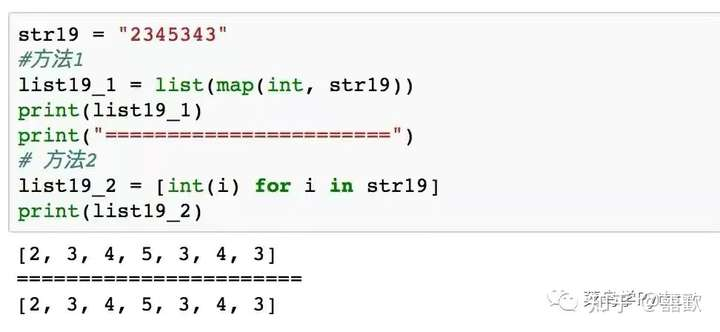## 14.使用try-except-finally模块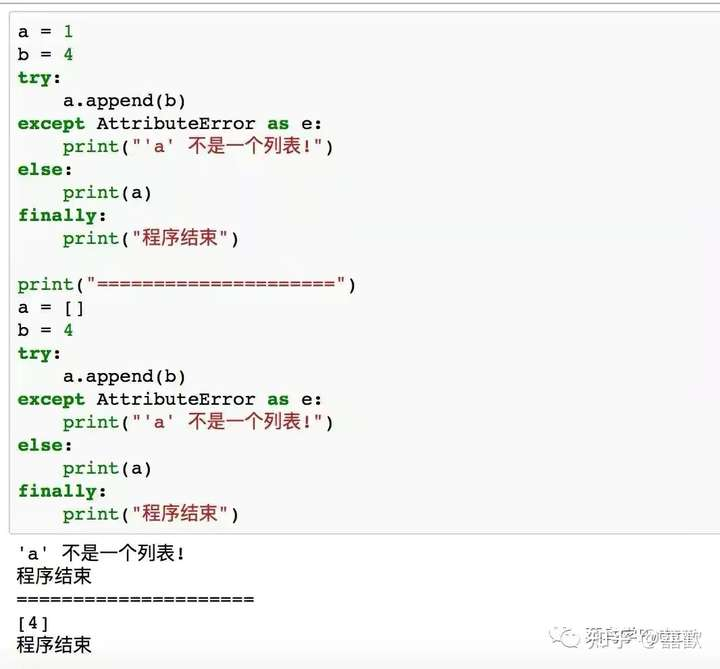## 15. 使用enumerate() 函数来获取索引-数值对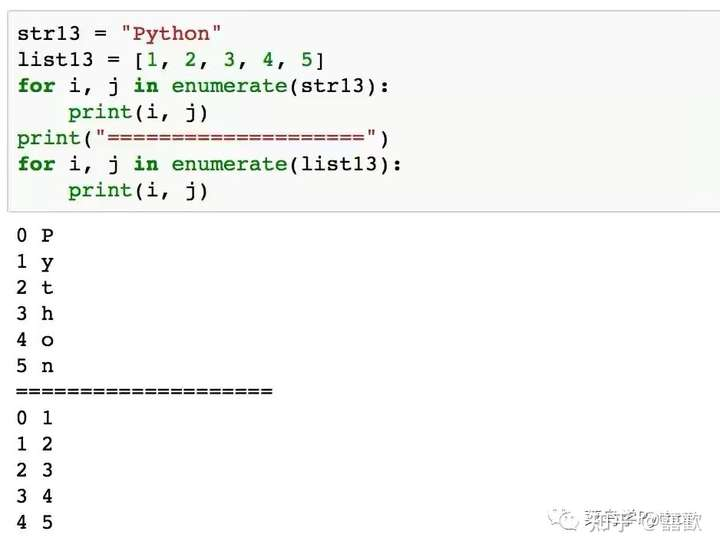enumerate() 函数用于将一个可遍历的数据对象(如上图的列表，字符串)组合为一个索引序列。

## 16.代码执行消耗时间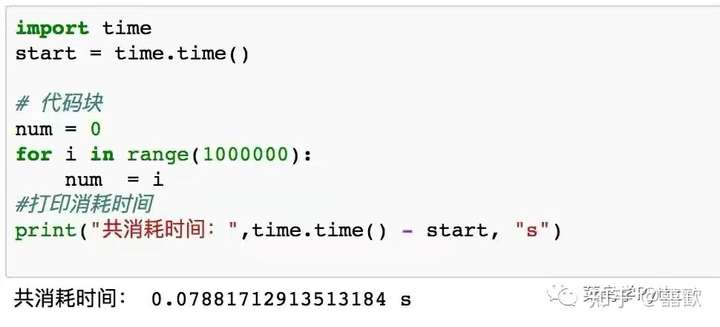## 17.检查对象的内存占用情况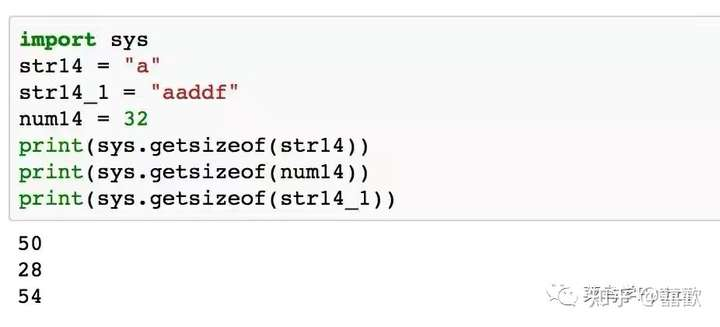## 18.字典的合并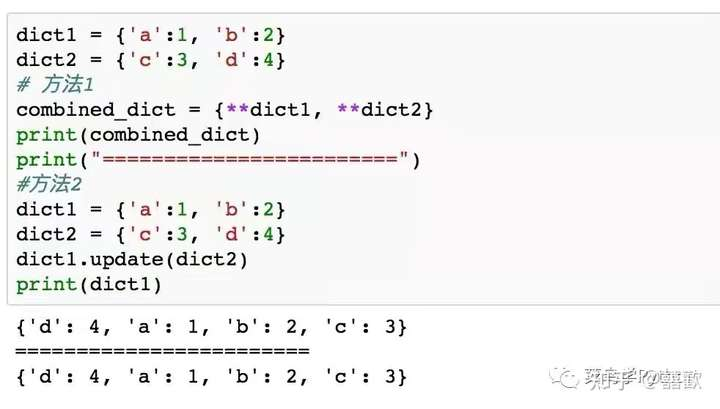## 19.随机采样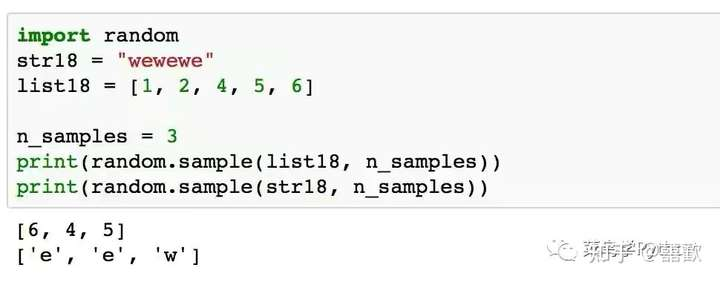## 20.检查唯一性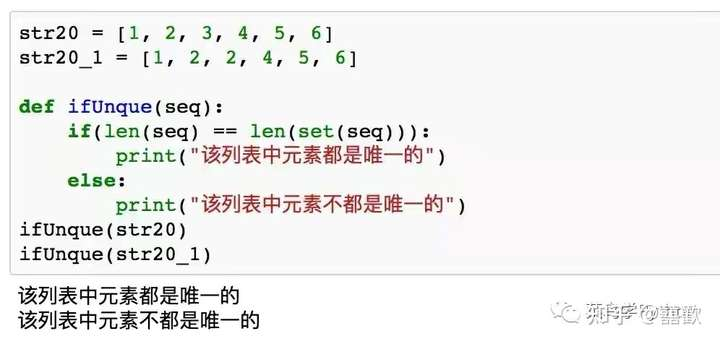# 8种高级的Python列表使用技巧，代码都给你整理好啦

from https://zhuanlan.zhihu.com/p/383464292 by liustar

Python中的列表是我们最常见的数据结构之一，也是最强大的数据结构之一！Python列表功能非常丰富，并且具有很多隐藏的技巧没有被发现。

## 一、使用Python过滤列表

1.使用Filter（）函数

filter（）函数采用两个参数：函数和可迭代项。 在这种情况下，我们将定义一个函数并过滤一个列表。

original_list = [1,2,3,4,5]

def filter_three(number):
return number > 3

filtered = filter(filter_three, original_list)

filtered_list = list(filtered)

print(filtered_list)
# Returns [4,5]


1. 我们定义了原始列表
2. 然后定义一个接受参数（“ number”）的函数。如果数字大于3，函数将返回True。
3. 我们定义了一个应用filter函数的项（“ filtered”）。我们的项已筛选，是筛选对象。
4. 最后，我们创建“ filtered_list”，将列表函数替换为filtered对象。

2.具有列表理解

original_list = [1,2,3,4,5]

filtered_list = [number for number in original_list if number > 3]

print(filtered_list)

# Return [4,5]


## 二、修改列表

1.使用Map（）函数

Python Map函数允许我们将函数应用于可迭代对象中的每个项。

original_list = [1,2,3,4,5]

def square(number):
return number ** 2

squares = map(square, original_list)

squares_list = list(squares)

print(squares)

# Returns [1, 4, 9, 16, 25]


2.具有列表理解

original_list = [1,2,3,4,5]

squares_list = [number ** 2 for number in original_list]

print(squares_list)

# Returns [1,4,9,16,25]


## 三、将列表与Zip（）函数合并

numbers = [1,2,3]

letters = ['a', 'b', 'c']

combined = zip(numbers, letters)

combined_list = list(combined)

# returns [(1, 'a'), (2, 'b'), (3, 'c')]


## 四、颠倒列表

Python列表是有序的数据结构。因此，项目的顺序很重要。有时我们可能需要颠倒列表中的项，这可以使用Python切片操作轻松完成。

original_list = [1,2,3,4,5]

reversed_list = original_list[::-1]

print(reversed_list)

# Returns: [5,4,3,2,1]


## 五、检查列表中的成员身份

games = ['Yankees', 'Yankees', 'Cubs', 'Blue Jays', 'Giants']

def isin(item, list_name):
if item in list_name: print(f"{item} is in the list!")
else: print(f"{item} is not in the list!")

isin('Blue Jays', games)
isin('Angels', games)

# Returns
#Blue Jays在名单上！
#Angels不在名单上！


## 六、查找列表中最常见的项

games = ['heads', 'heads', 'tails', 'heads', 'tails']
items = set(games)
print(max(items, key = games.count))


1. 我们用五个正面或反面游戏的结果来定义一个列表；
2. 我们定义了列表中所有项的集合。set（）函数过滤掉了列表中的重复项；
3. 最后，我们将max（）应用于项目集，并使用key参数作为集合中元素的最高计数。

## 七、展开列表

nested_list = [[1,2,3],[4,5,6],[7,8,9]]

flat_list = [i for j in nested_list for i in j]

print(flat_list)

# Returns [1, 2, 3, 4, 5, 6, 7, 8, 9]


## 八、检查唯一性

Python中的集合类似于列表（因为它是可变且无序的），但是它只能包含唯一的项。

list1 = [1,2,3,4,5]
list2 = [1,1,2,3,4]

def isunique(list):
if len(list) == len(set(list)):
print('Unique!')
else: print('Not Unique!')

isunique(list1)
isunique(list2)

# Returns
# 唯一性
# 不唯一


# Python优雅写法，让你工作效率翻2倍

fromhttps://zhuanlan.zhihu.com/p/66488546 by Wayne

### 常规方法：

a = 0
b = 1
c = 2


### 优雅方法：

a, b, c = 0, 1, 2


### 常规方法：

info = ['brucepk', 'man', 'python']
name = info
sex = info
tech = info
print(name,sex,tech)

# 结果
brucepk man python


### 优雅方法：

info = ['brucepk', 'man', 'python']
name,sex,tech = info
print(name,sex,tech)

# 结果
brucepk man python


### 常规方法：

x = -6
if x < 0:
y = -x
else:
y = x
print(y)

# 结果
6


### 优雅方法：

x = -6
y = -x if x<0 else x
print(y)

# 结果
6


### 常规方法：

score = 82
if score >=80 and score < 90:
level = 'B'
print(level)

# 结果
B


### 优雅方法：

score = 82
if  80 <= score < 90:
level = 'B'
print(level)

# 结果
B


### 常规方法：

num = 1
if num == 1 or num == 3 or num == 5:
type = '奇数'
print(type)

# 结果



### 优雅方法：

num = 1
if num in(1,3,5):
type = '奇数'
print(type)

# 结果



### 常规方法：

A,B,C =[1,3,5],{},''
if len(A) > 0:
print('A 为非空')
if len(B) > 0:
print('B 为非空')
if len(C) > 0:
print('C 为非空')

# 结果
A 为非空


### 优雅方法：

A,B,C =[1,3,5],{},''
if A:
print('A 为非空')
if B:
print('B 为非空')
if C:
print('C 为非空')

# 结果
A 为非空


### 常规方法：

math,English,computer =90,80,88
if math<60 or English<60 or computer<60:
print('not pass')

# 结果
not pass


### 优雅方法：

math,English,computer =90,59,88
if any([math<60,English<60,computer<60]):
print('not pass')

# 结果
not pass


### 常规方法：

math,English,computer =90,80,88
if math>60 and English>60 and computer>60:
print('pass')

# 结果
pass


### 优雅方法：

math,English,computer =90,80,88
if all([math>60,English>60,computer>60]):
print('pass')

# 结果
pass


### 常规方法：

L =['math', 'English', 'computer', 'Physics']
for i in range(len(L)):
print(i, ':', L[i])

# 结果
0 : math
1 : English
2 : computer
3 : Physics


### 优雅方法：

L =['math', 'English', 'computer', 'Physics']
for k,v in enumerate(L):
print(k, ':', v)

# 结果
0 : math
1 : English
2 : computer
3 : Physics


### 常规方法：

L = []
for i in range(1, 6):
L.append(i*i)
print(L)

#结果：
[1, 4, 9, 16, 25]


### 优雅方法：

print([x*x for x in range(1, 6)])

#结果：
[1, 4, 9, 16, 25]


Python 这些优雅的写法学会了吗？自己赶紧动手试试吧。

# 9个实用的Python小技巧，让你编写出更快、更好的脚本!

from https://zhuanlan.zhihu.com/p/380482726

，专注Python、数据分析、数据挖掘、好玩工具

### 1、使用defaultdict和lambda函数创建字典

from collections import defaultdict
import numpy as np
q = defaultdict(lambda: np.zeros(5))
# Example output
In : q
Out: array([0., 0., 0., 0., 0.])


defaultdicts 最酷的一点是，它们永远不会引发 KeyError 。任何不存在的键都会获取默认工厂返回的值，在本例中，默认工厂是一个lambda函数，它为给定的任何键返回一个默认NumPy数组，其中包含5个零。

### 2、正则表达式基本配方

import re
pattern = re.compile(r”\d\d”)
print(re.search(pattern,"Let's find the number 23").group())
# or
print(re.findall(pattern, “Let's find the number 23”))
# Outputs
'23'
'23'


Regex 对于许多 python 程序员来说都是必须的，所以记住核心Regex方法总是很有帮助的。

### 3、使用集合从两个列表中获得差异

list1 = [1,2,3,4,5]
list2 = [3,4,5]
print(list(set(list1) — set(list2)))
# or
print(set(lista1).difference(set(lista2)))
# Outputs
[1,2]
{1,2}


### 4、partial 函数

from functools import partial
def multiply(x,y):
return x*y
dbl = partial(multiply,2)
print(dbl)
print(dbl(4))
# Outputs

functools.partial(<function multiply at 0x7f16be9941f0>, 2)
8


### 5、使用 hasattr（）内置方法获取object属性

class SomeClass:
def __init__(self):
self.attr1 = 10
def attrfunction(self):
print("Attreibute")
hasattr(SomeClass, "attrfunction")
# Output
True


### 6、使用isinstance（）检查变量是否为给定类型

isinstance(1, int)
#Output
True


### 7、使用map（）打印列表中的数字

list1 = [1,2,3]
list(map(print, list1))
# Output
1
2
3


### 8、使用.join（）方法格式化datetime日期

from datetime import datetime
date = datetime.now()
print("-".join([str(date.year), str(date.month), str(date.day)])
# Output
'2021-6-15'


### 9、将两个具有相同规则的列表随机化

import numpy as np
x = np.arange(100)
y = np.arange(100,200,1)
idx = np.random.choice(np.arange(len(x)), 5, replace=False)
x_sample = x[idx]
y_sample = y[idx]
print(x_sample)
print(y_sample)
# Outputs
array([68, 87, 41, 16,  0])
array([168, 187, 141, 116, 100])


## 文章推荐

Python 自动化 Excel ？这15个技巧要会！

4 款 Python 数据探索性分析(EDA)工具包，总有一款适合你！kerbal